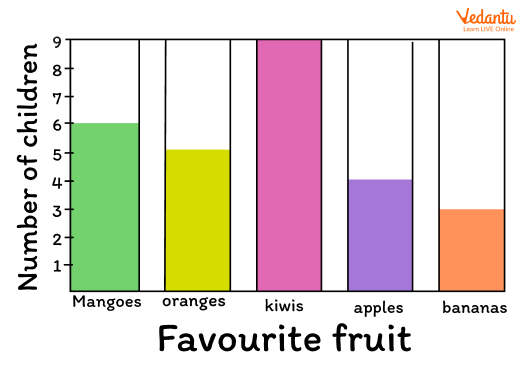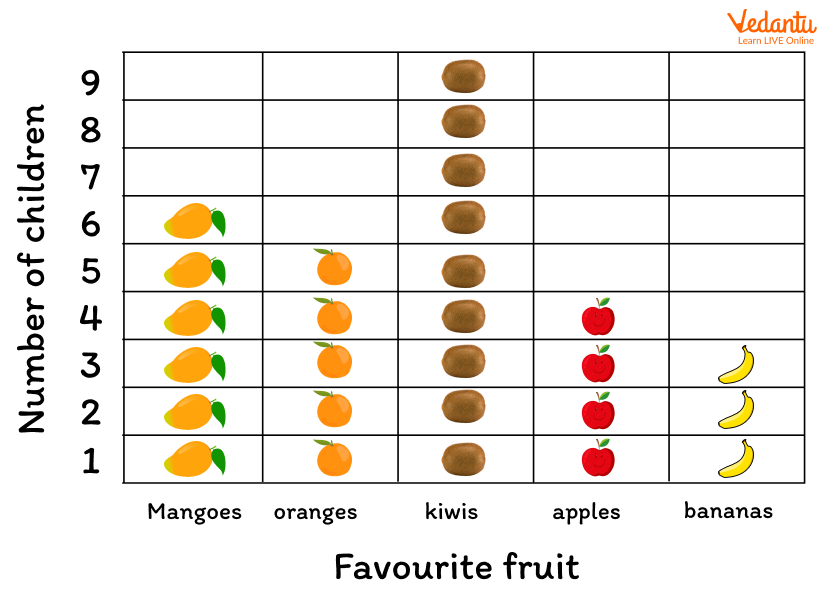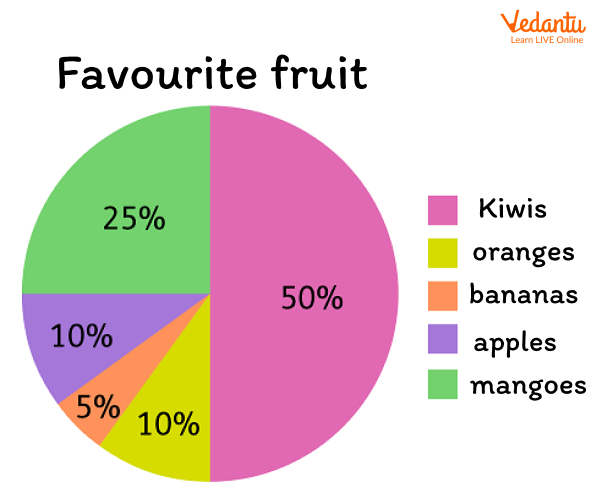Courses
Courses for Kids
Free study material
Free LIVE classes
More

# Statistics in Year 3 (Age-Group 7–8)## An Introduction to Year 3 Statistics

Do you know what statistics is? Statistics is a branch of Mathematics where we collect, analyse, understand and represent data. What is data? It is a collection of information, could be numbers, facts, etc., that is assembled through measurement, questioning or observing. Data in statistics are collected through experiments and surveys. It is used in different fields for dealing with data and is helpful for storing information in simple form.

Children are able to identify and interpret different types of graphs at the age of 7 to 8 years. In this article, we will learn about statistics for kids who are in the learning phase of year 3.

## How Can You Represent Data in Statistics?

Data can be presented in different ways. Some main methods of data presentation are given below.

1. Bar Graph

A bar chart is used to represent data which is distributed such as the number of students in a class, number of fruits in a basket, etc. Hence, a bar graph is used where data can only have a limited number of possible values. In a bar chart, data is represented by the lengths of a set of bars. Some gaps are usually present between the bars. The bars in such graphs can be drawn vertically or horizontally. For example, a bar graph of the number of children vs favourite fruit is drawn below. In the following bar graph, data of different fruits are drawn as bars.Example of Bar Graph

2. Pictogram

A pictogram is also used for the representation of data in statistics. It is also known as pictographs or picture graphs. Pictures are used in pictograms to show quantities. A picture is used to show one thing or a number of things. The same example of the number of children vs favourite fruit example is shown below in the form of a presentation of a pictogram. Here, pictures are used to represent the quantities, that is, which fruit is most liked by the maximum number of children.Example of Pictogram

3. Pie Chart

Data or information is represented in a circle in a pie chart. The slices in a pie chart or different-sized sectors of the circle stand for the different quantities they represent. For example, the slices of a pie chart can represent the percentage of the children who like different fruits. If the same example of the number of children and their favourite fruits data is drawn in the form of a pie chart, it will look like the following figure. The one with the highest percentage or great area of slice has the most liked fruit.Example of Pie- Chart

## The Collection of Information Through Data

Different types of information can be gathered from bar graphs, pie charts, pictographs etc. Interpretation of data is also an important part of statistical analysis. It provides useful information that has been stored in the form of bars and graphs. In the above example, the favourite fruits of children are analysed such as what percentage of kids like apples, bananas, oranges, grapes and apples.

## Conclusion

Statistics is used where data is important to store in an easy way. The above article is useful for kids in order to understand the concept of statistics. Bar graph, pictograph and pie chart is discussed in the article with help of examples.

Last updated date: 20th Sep 2023
Total views: 132.9k
Views today: 1.32k

## FAQs on Statistics in Year 3 (Age-Group 7–8)

1. What is the need for statistics?

In statistics, large and complex data is stored in an easy method so that we can interpret data as per our requirements. If we do not do statistical analysis the data will be complex and getting correct information will be a challenging task. Hence, we need statistics to store data that we can decode easily.

2. What are kids required to know about in Year 3 statistics?

Kids of age group 7-8 years are required to know how data is presented in statistics and how the data is interpreted from bar graphs, pictographs and pie charts. They should understand the tabular representation of data.